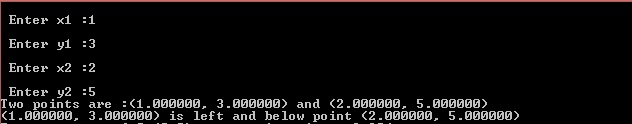# Answered! Programming Assignment Write a program position.cpp that reads two points and reports if the first point is above/below/left/right or equals the second point….

Programming Assignment
Write a program position.cpp that reads two points and reports if the first point is
above/below/left/right or equals the second point.
1. Type “emacs position.cpp &“ (without the double quotes) at the terminal to create a
new file, position.cpp.
2. Prompt and read in the (two) coordinates, (x1, y1), of the first point. The coordinates are not
necessarily integers.
3. Prompt and read in the (two) coordinates, (x2, y2), of the second point. The coordinates are
not necessarily integers.
4. Print out one of the following messages depending on the relative location of the first and
second points.
 Point (x1, y1) is left and below point (x2, y2).
 Point (x1, y1) is left and above point (x2, y2).
 Point (x1, y1) is left of (x2, y2).
 Point (x1, y1) is right and below point (x2, y2).
 Point (x1, y1) is right and above point (x2, y2).
 Point (x1, y1) is right of point (x2, y2).
 Point (x1, y1) is below point (x2, y2).
 Point (x1, y1) is above point (x2, y2).
 Point (x1, y1) equals point (x2, y2).
(Replace (x1, y1) and (x2, y2) with the actual coordinates of the first and second points,
respectively.)
Point (x1, y1) is left and below point (x2, y2) if x1 < x2 and y1 < y2.
Point (x1, y1) is left and above point (x2, y2) if x1 < x2 and y1 > y2.
Point (x1, y1) is left of point (x2, y2) if x1 < x2 and y1 = y2.
Point (x1, y1) is below point (x2, y2) if x1 = x2 and y1 < y2.
Similar relationships hold for the other outputs.

Solution :

Code :

Don't use plagiarized sources. Get Your Custom Essay on
Answered! Programming Assignment Write a program position.cpp that reads two points and reports if the first point is above/below/left/right or equals the second point….
GET AN ESSAY WRITTEN FOR YOU FROM AS LOW AS \$13/PAGE

#include <stdio.h>
int main(void)
{
float x1, y1, x2, y2;
printf(“n Enter x1 :”);
scanf(“%f”, &x1);
printf(“n Enter y1 :”);
scanf(“%f”, &y1);
printf(“n Enter x2 :”);
scanf(“%f”, &x2);
printf(“n Enter y2 :”);
scanf(“%f”, &y2);

printf(“Two points are :(%f, %f) and (%f, %f)n”, x1,y1,x2,y2);
if(x1 < x2 && y1 < y2){
printf(“(%f, %f) is left and below point (%f, %f)”, x1, y1, x2,y2);
}
else if(x1 < x2 && y1 > y2){
printf(“(%f, %f) is left and above point (%f, %f)”, x1, y1, x2,y2);
}
else if(x1 < x2 && y1 == y2){
printf(“(%f, %f) is left of point (%f, %f)”, x1, y1, x2,y2);
}
else if(x1 > x2 && y1 > y2){
printf(“(%f, %f) is right and above of point (%f, %f)”, x1, y1, x2,y2);
}
else if(x1 > x2 && y1 < y2){
printf(“(%f, %f) is right and below of point (%f, %f)”, x1, y1, x2,y2);
}
else if(x1 > x2 && y1 == y2){
printf(“(%f, %f) is right of point (%f, %f)”, x1, y1, x2,y2);
}
else if(x1 == x2 && y1 > y2){
printf(“(%f, %f) is above point (%f, %f)”, x1, y1, x2,y2);
}
else if(x1 == x2 && y1 < y2){
printf(“(%f, %f) is below point (%f, %f)”, x1, y1, x2,y2);
}
else if(x1 == x2 && y1 == y2){
printf(“(%f, %f) equals point (%f, %f)”, x1, y1, x2,y2);
}
return 0;
}

Output :